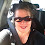43
Explanation
First Equation,
10 + 10 + 10 = 30

Second Equation,
5 + 5 + 10 = 20

Third Equation,
4 + 4 + 5 = 13

So Single shoe is 10/2 = 5
Man = 5
Single Paper corn = 2

Final equation becomes,
5 +  (5+4+10) x 2 = 43 (In man's hand there is two paper corns and he is wearing those rose shoe pair)

Get More WhatsApp Puzzles with Answer

1.I think 43 is correct answer because 5+(5+4+10)×2

1.Yes 43 is correct.

2.how is it came 43

2.Maybe am different, i can't accept when you divide the shoes, its not the same shoes like paper corn, its two different sides of shoes, so I can say, its 1 and 0 which came 10, left shoe is 1, right shoe is 0, and did you think why that paper corn at the end is little sliding the right side?

3.multiply first and each item
500x2+5= 1005

4.Sorry but I dont agree. There is no any logic that in there is a + symbol for the man and popcorns and shoes. the picture can be undestood as: 5(man)4(corns)10(shoes) or 5410. So the answer can be:
5+5410x2 = 10825.

5.6.This comment has been removed by the author.

7.The picture is deceiving. In the first few equations, it appears that the boy is carrying books. This misleads people in thinking that what the boy is carrying determines the number.

8.What about the black shoes he's wearing?

9.but the man (5) was holding 2 paper corns (2 and 2) so 4 has to be included in this

10.Some digital sellers may not refund your money but they can allow you to choose another pair of shoes. scarpe uomo estive